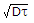Electronics and Communication Engineering - Electronic Devices and Circuits

46.

A p-n junction diode has

 A. low forward and high reverse resistance B. a non-linear v-i characteristics C. zero forward current till the forward voltage reaches cut in value D. all of the above

Explanation:

A p-n Junction has all these features.

47.

Which of the following is true as regards photo emission?

 A. Velocity of emitted electrons is dependent on light intensity B. Rate of photo emission is inversely proportional to light intensity C. Maximum velocity of electron increases with decreasing wave length D. Both holes and electrons are produced

Explanation:

As wavelength decreases, frequency increases and maximum velocity of electron increases.

48.

The power dissipation in a transistor is the product of

 A. emitter current and emitter to base voltage B. emitter current and collector to emitter voltage C. collector current and collector to emitter voltage D. none of the above

Explanation:

Maximum power dissipation occurs at collector junction.

49.

The normal operation of JFET is

 A. constant voltage region B. constant current region C. both constant voltage and constant current regions D. either constant voltage or constant current region

Explanation:

In major portion of drain characteristics ID is constant.

50.

The minority carrier life time and diffusion constant in a semiconductor material are respectively 100 microsecond and 100 cm2/sec. The diffusion length is

 A. 0.1 cm B. 0.01 cm C. 0.0141 cm D. 1 cm

Diffusion length =.